Courses

# DC Generators Notes | EduRev

## Electrical Engineering (EE) : DC Generators Notes | EduRev

The document DC Generators Notes | EduRev is a part of the Electrical Engineering (EE) Course Basic Electrical Technology.
All you need of Electrical Engineering (EE) at this link: Electrical Engineering (EE)

Goals of the lesson

Students are expected to learn about the classification of d.c generators and their characteristics in this lesson.  After going through this lesson they will be able to:

1. differentiate different types of d.c generators along with their schematic representations.
2. identify, looking at the circuit diagram of a given generator, the types of the generator i.e., whether the generator is shunt, separately excited or compound generator.
3. sketch the important characteristics (no load and load characteristics) of different types of generators.
4. identify various factors responsible for internal voltage drop.
5. understand the conditions to be fulfilled so as to build up voltage in a shunt generator.
6. predict the load characteristic of a shunt generator from its open circuit characteristic.
7. locate the shunt and series field coils in the machine and comment on their relative number of turns and cross sectional areas.

Generator types & Characteristics

D.C generators may be classified as (i) separately excited generator, (ii) shunt generator, and (iii) series generator and (iv) compound generator.  In a separately excited generator field winding is energised from a separate voltage source in order to produce flux in the machine. So long the machine operates in unsaturated condition the flux produced will be proportional to the field current. In order to implement shunt connection, the field winding is connected in parallel with the armature. It will be shown that subject to fulfillment of certain conditions, the machine may have sufficient field current developed on its own by virtue of its shunt connection.

In series d.c machine, there is one field winding wound over the main poles with fewer turns and large cross sectional area. Series winding is meant to be connected in series with the armature and naturally to be designed for rated armature current. Obviously there will be practically no voltage or very small voltage due to residual field under no load condition (Ia = 0). However, field gets strengthened as load will develop rated voltage across the armature with reverse polarity, is connected and terminal voltage increases. Variation in load resistance causes the terminal voltage to vary. Terminal voltage will start falling, when saturation sets in and armature reaction effect becomes pronounced at large load current. Hence, series generators are not used for delivering power at constant voltage. Series generator found application in boosting up voltage in d.c transmission system.

A compound generator has two separate field coils wound over the field poles. The coil having large number of turns and thinner cross sectional area is called the shunt field coil and the other coil having few number of turns and large cross sectional area is called the series field coil. Series coil is generally connected in series with the armature while the shunt field coil is connected in parallel with the armature. If series coil is left alone without any connection, then it becomes a shunt machine with the other coil connected in parallel. Placement of field coils for shunt, series and compound generators are shown in figure 38.1.  Will develop rated voltage across the armature with reverse polarity.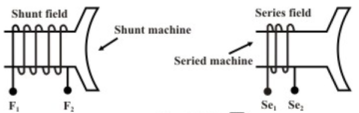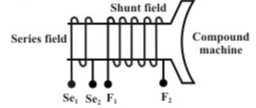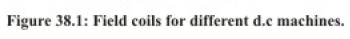Characteristics of a separately excited generator

No load or Open circuit characteristic

In this type of generator field winding is excited from a separate source, hence field current is independent of armature terminal voltage as shown on figure (38.2). The generator is driven by a prime mover at rated speed, say n rps. With switch S in opened condition, field is excited via a potential divider connection from a separate d.c source and field current is gradually increased. The field current will establish the flux per pole φ. The voltmeter V connected across the armature terminals of the machine will record the generated emf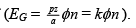Remember pz/a  is a constant (k) of the machine. As field current is increased, EG will increase. EG versus If plot at constant speed n is shown in figure (38.3a).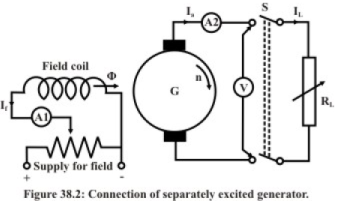It may be noted that even when there is no field current, a small voltage (OD) is generated due to residual flux. If field current is increased, φ increases linearly initially and O.C.C follows a straight line. However, when saturation sets in, φ practically becomes constant and hence Eg too becomes constant. In other words, O.C.C follows the B-H characteristic, hence this characteristic is sometimes also called the magnetisation characteristic of the machine.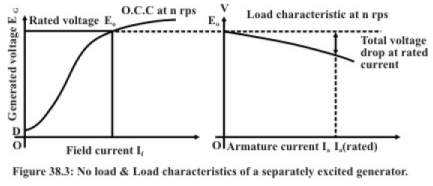It is important to note that if O.C.C is known at a certain speed nl, O.C.C at another speed n2 can easily be predicted. It is because for a constant field current, ratio of the generated voltages becomes the ratio of the speeds as shown below.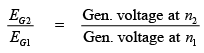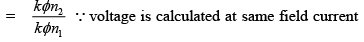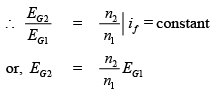Therefore points on O.C.C at n2 can be obtained by multiplying ordinates of O.C.C at n1 with the ratio n2/n1 O.C.C at two different speeds are shown in the following figure (38.4).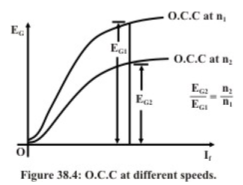Load characteristic of separately excited generator

Load characteristic essentially describes how the terminal voltage of the armature of a generator changes for varying armature current Ia. First at rated speed, rated voltage is generated across the armature terminals with no load resistance connected across it (i.e., with S opened) by adjusting the field current.  So for Ia = 0, V = Eo should be the first point on the load characteristic. Now with S is closed and by decreasing RL from infinitely large value, we can increase Igradually and note the voltmeter reading. Voltmeter reads the terminal voltage and is expected to decrease due to various drops such as armature resistance drop and brush voltage drop. In an uncompensated generator, armature reaction effect causes additional voltage drop. While noting down the readings of the ammeter A2 and the voltmeter V, one must see that the speed remains constant at rated value. Hence the load characteristic will be drooping in nature as shown in figure (38.3b)

Characteristics of a shunt generator

We have seen in the previous section that one needs a separate d.c supply to generate d.c voltage. Is it possible to generate d.c voltage without using another d.c source? The answer is yes and for obvious reason such a generator is called self excited generator.

Field coil (F1, F2) along with a series external resistance is connected in parallel with the armature terminals (A1, A2) of the machine as shown in figure (38.5). Let us first qualitatively explain how such connection can produce sufficient voltage. Suppose there exists some residual field. Therefore, if the generator is driven at rated speed, we should expect a small voltage (kφresn) to be induced across the armature. But this small voltage will be directly applied across the field circuit since it is connected in parallel with the armature. Hence a small field current flows producing additional flux. If it so happens that this additional flux aids the already existing residual flux, total flux now becomes more generating more voltage. This more voltage will drive more field current generating more voltage. Both field current and armature generated voltage grow cumulatively.

This growth of voltage and the final value to which it will settle down can be understood by referring to (38.6) where two plots have been shown. One corresponds to the O.C.C at rated speed and obtained by connecting the generator in separately excited fashion as detailed in the preceding section. The other one is the V-I characteristic of the field circuit which is a straight line passing through origin and its slope represents the total field circuit resistance.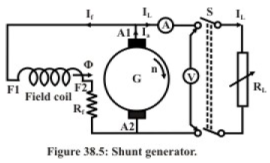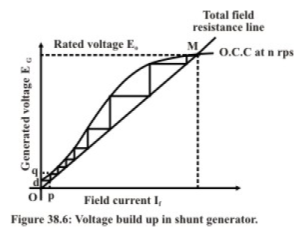Initially voltage induced due to residual flux is obtained from O.C.C and given by Od. The field current thus produced can be obtained from field circuit resistance line and given by Op. In this way voltage build up process continues along the stair case. The final stable operating point (M) will be the point of intersection between the O.C.C and the field resistance line. If field circuit resistance is increased, final voltage decreases as point of intersection shifts toward left. The field circuit resistance line which is tangential to the O.C.C is called the critical field resistance. If the field circuit resistance is more than the critical value, the machine will fail to excite and no voltage will be induced - refer to figure 38.7. The reason being no point of intersection is possible in this case.

Suppose a shunt generator has built up voltage at a certain speed. Now if the speed of the prime mover is reduced without changing Rf, the developed voltage will be less as because the O.C.C at lower speed will come down (refer to figure 38.8). If speed is further reduced to a certain critical speed (ncr), the present field resistance line will become tangential to the O.C.C at ncr. For any speed below ncr, no voltage built up is possible in a shunt generator.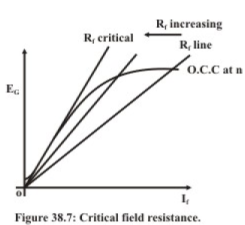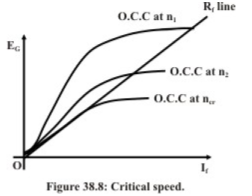A shunt generator driven by a prime mover, can not built up voltage if it fails to comply any of the conditions listed below.

1. The machine must have some residual field. To ensure this one can at the beginning excite the field separately with some constant current. Now removal of this current will leave some amount of residual field.
2. Field winding connection should be such that the residual flux is strengthened by the field current in the coil. If due to this, no voltage is being built up, reverse the field terminal connection.
3. Total field circuit resistance must be less than the critical field resistance.

EG1 −V1 = lengthPQ

= 1a1ra + brush drop + drop due to armature reaction

EG1−V1 ≈ Ia1ra neglecting brush drop & armature reaction drop,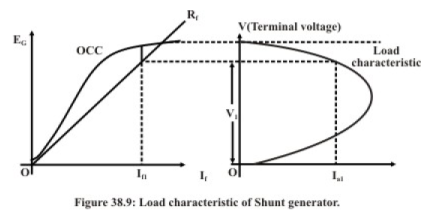Prediction of approximate load characteristic from OCC & Rline

We have seen in the preceding section that for a particular load current Ia, the terminal voltage V and the generated voltage EG are related by EG −V ≈ Ia ra . This relationship along with OCC and Rf line can be used to predict the load characteristic. This can be done in following two ways.

First way

2. Choose a particular value of V( < Eo). The idea is to know which Ia has changed the terminal voltage to V. Refer to figure (38.10).
3. Now draw a horizontal line NQ which intersects the field resistance line at Q.
4. Draw a vertical line PQ intersecting OCC at P.
5. Length PQ must be I a ra drop.
6. Knowing the value of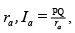can be calculated.
7. The above steps are repeated, for other values of chosen V.
8. Plot of all these pair of [V, Ia] will give the load characteristic.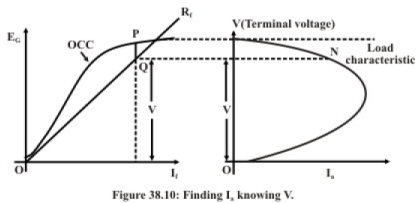Alternative method

In this method which is also graphical, we try to estimate the terminal voltage V for a given armature current Ia. Following steps may be adopted for the purpose.

1. Choose a particular value of armature current Ia.
2. Since armature resistance is known, calculate I a ra .
3. Mark a point K on the voltage axis of OCC such that OK = Ia ra .
4. Draw a line parallel to Rf line and passing through point K. This line in general expected to intersect the OCC at two points G and H as shown in figure (38.11).
5. Draw two horizontal lines passing through G and H respectively.
6. Draw a vertical line in load characteristic plane at the chosen current Ia. This vertical line intersect the two horizontal lines drawn in the previous step at points P & Q respectively.
7. Thus we find the generator can deliver the chosen Ia at two different terminal voltages of Vp and VQ. So the load characteristic is a double valued function.
8. It should be noted that, there exists a line parallel to the Rf line which touches the OCC only at one point S as shown. For this we shall get a single terminal voltage as represented by the point T. The corresponding armature current (I a max) is the maximum .value which the generator is capable of delivering.
9. In fact it explains why the load characteristic takes a turn toward left after reaching a maximum current.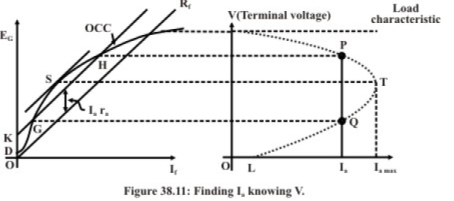As we go on decreasing the load resistance, terminal voltage falls. Field being connected across the armature, φ also falls reducing the generated voltage EG which was not the case with separately excited generator where φ produced was independent of the terminal voltage of the generator. Thus after reaching Ia max, any further decrement in the load resistance causes EG to fall substantially to make Ia < Ia max, In fact, If we reduce RL to the extent that RL = 0 (i.e., putting a short circuit across the terminals), field current becomes zero making EG = residual voltage = OD. Therefore steady short circuit current of the generator will be pretty small and equal to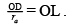Compound generator

As introduced earlier, compound machines have both series and shunt field coils. On each pole these two coils are placed as shown in figure 38.1. Series field coil has low resistance, fewer numbers of turns with large cross sectional area and connected either in series with the armature or in series with the line. On the other hand shunt field coil has large number of turns, higher resistance, small cross sectional area and either connected in parallel across the armature or connected in parallel across the series combination of the armature and the series field. Depending on how the field coils are connected, compound motors are classified as short shunt and long shunt types as shown in figures 38.12 and 38.13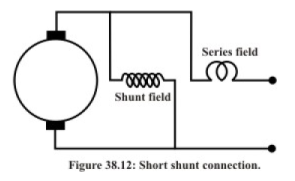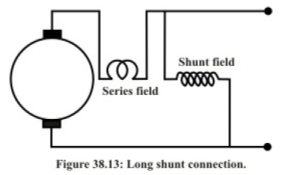Series field coil may be connected in such a way that the mmf produced by it aids the shunt field mmf-then the machine is said to be cumulative compound machine, otherwise if the series field mmf acts in opposition with the shunt field mmf – then the machine is said to be differential compound machine.

In a compound generator, series field coil current is load dependent. Therefore, for a cumulatively compound generator, with the increase of load, flux per pole increases. This in turn increases the generated emf and terminal voltage. Unlike a shunt motor, depending on the strength of the series field mmf, terminal voltage at full load current may be same or more than the no load voltage. When the terminal voltage at rated current is same that at no load condition, then it is called a level compound generator. If however, terminal voltage at rated current is more than the voltage at no load, it is called a over compound generator. The load characteristic of a cumulative compound generator will naturally be above the load characteristic of a shunt generator as depicted in figure 38.14. At load current higher than the rated current, terminal voltage starts decreasing due to saturation, armature reaction effect and more drop in armature and series field resistances.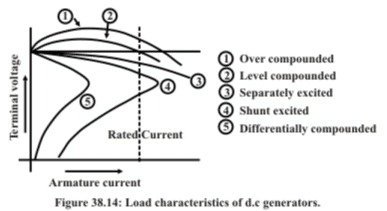To understand the usefulness of the series coil in a compound machine let us undertake the following simple calculations. Suppose as a shunt generator (series coil not connected) 300 AT/pole is necessary to get no load terminal voltage of 220 V. Let the terminal voltage becomes 210 V at rated armature current of 20 A. To restore the terminal voltage to 220 V, shunt excitation needs to be raised such that AT/pole required is 380 at 20 A of rated current. As a level compound generator, the extra AT (380-300 = 80) will be provided by series field. Therefore, number of series turns per pole will be 80/20 = 4. Thus in a compound generator series field will automatically provide the extra AT to arrest the drop in terminal voltage which otherwise is inevitable for a shunt generator.

For the differentially compounded generator where series field mmf opposes the shunt field mmf the terminal voltage decreases fast with the increase in the load current.

Offer running on EduRev: Apply code STAYHOME200 to get INR 200 off on our premium plan EduRev Infinity!

57 docs|59 tests

,

,

,

,

,

,

,

,

,

,

,

,

,

,

,

,

,

,

,

,

,

;